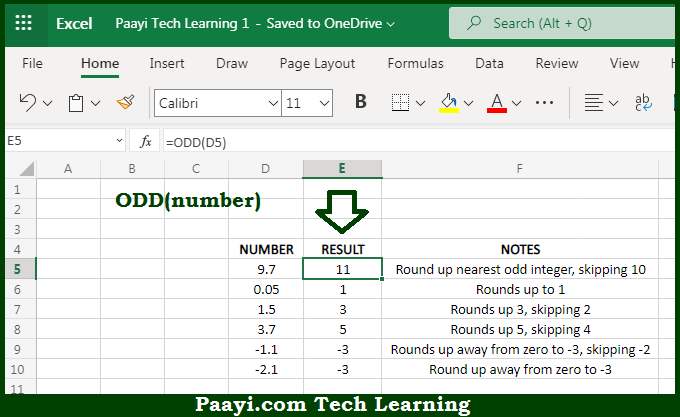# Learn How to Use Microsoft Excel ODD Function

Written by | 0 Comments | 592 Views

In this article, you will learn how to use the Microsoft Excel ODD function and its prime function in Microsoft Excel. You will also get to know the Microsoft Excel ODD function return value and syntax with the help of some examples.

Microsoft Excel ODD Function

The main function of the Microsoft Excel ODD function is to round a number up to the next odd integer. That implies, with the help of the ODD function you can able to return the next odd integer after rounding up the given number. It should be noted that the ODD function always rounds the number up, which is away from zero. That results in positive numbers becoming larger and negative numbers smaller or more negative. So, with the help of the ODD function, you can able to get the next odd integer after rounding.

Return Value of ODD Function

The return value will be the rounding number.

Syntax of ODD Function

=ODD(number)

Where the arguments:

• number: This is the number you want to round up to an odd integer.

## How to Use Microsoft Excel ODD Function?So we know that Microsoft Excel ODD function you can able to round a number up to the next odd integer. That implies, with the help of the ODD function you can able to return the next odd integer after rounding up the given number. It should be noted that the ODD function always rounds the number up, which is away from zero. That results in positive numbers becoming larger and negative numbers smaller or more negative. So, with the help of the ODD function, you can able to get the next odd integer after rounding.